Perpendicular bisector of a line segment

Practical Geometry
Concept wise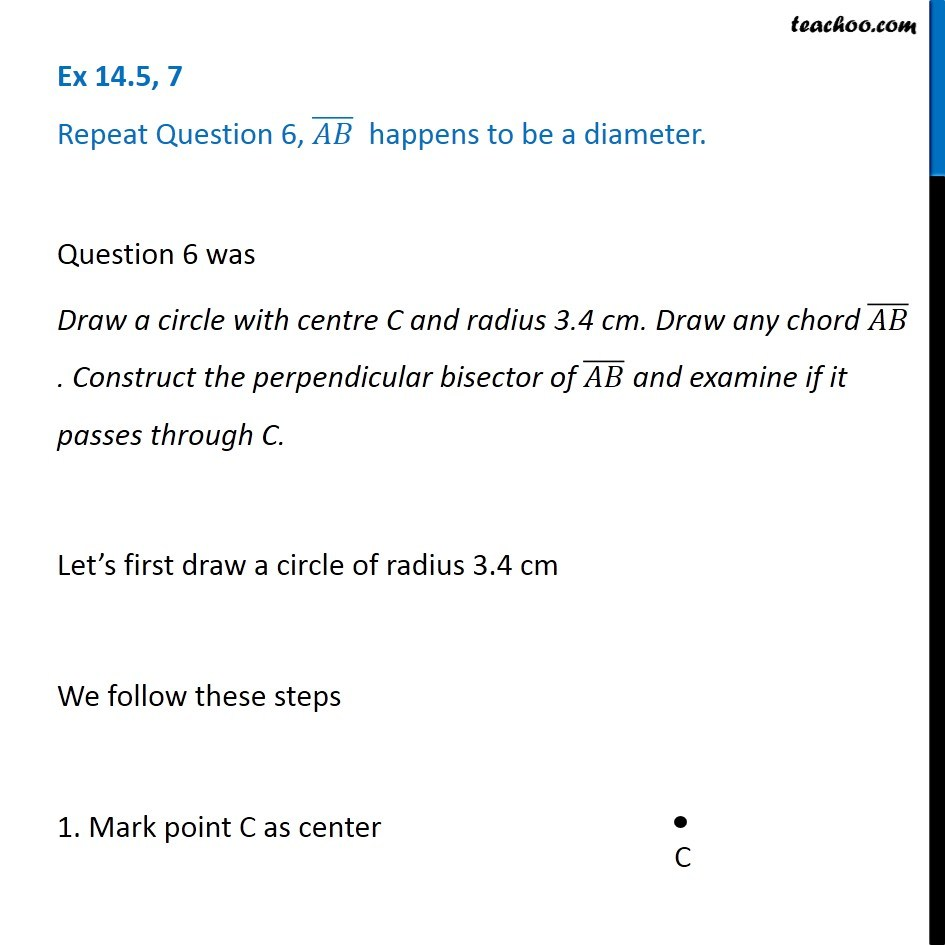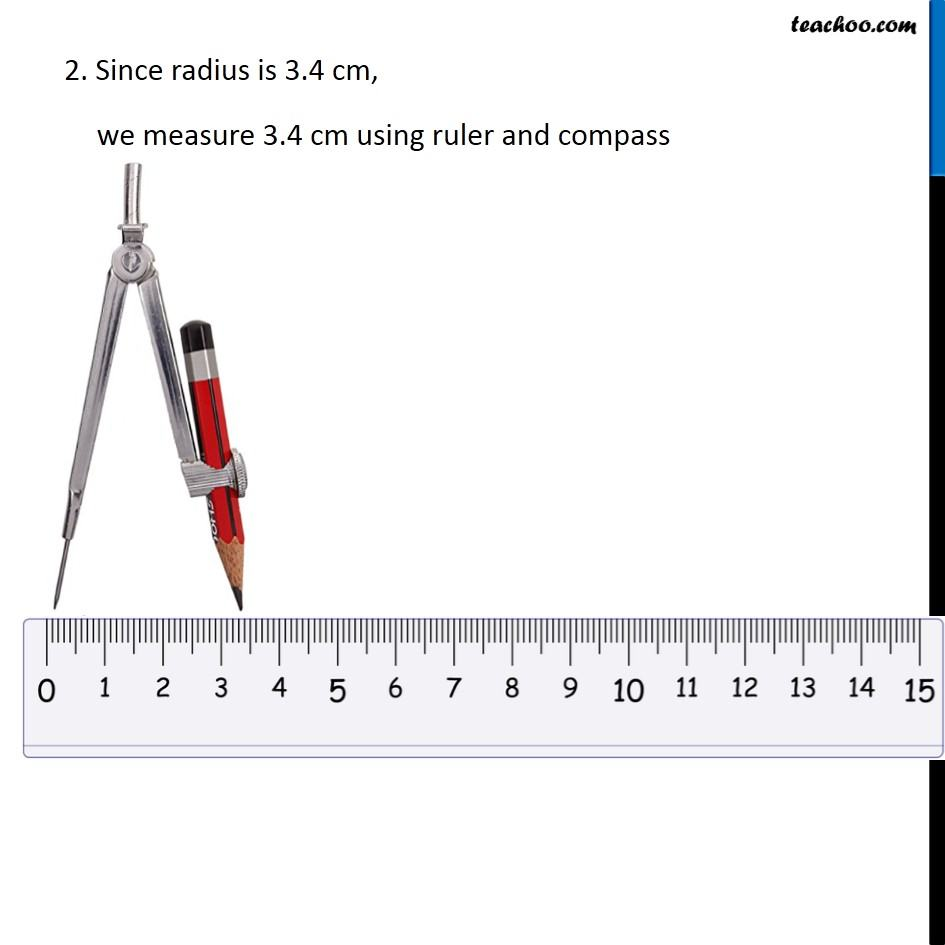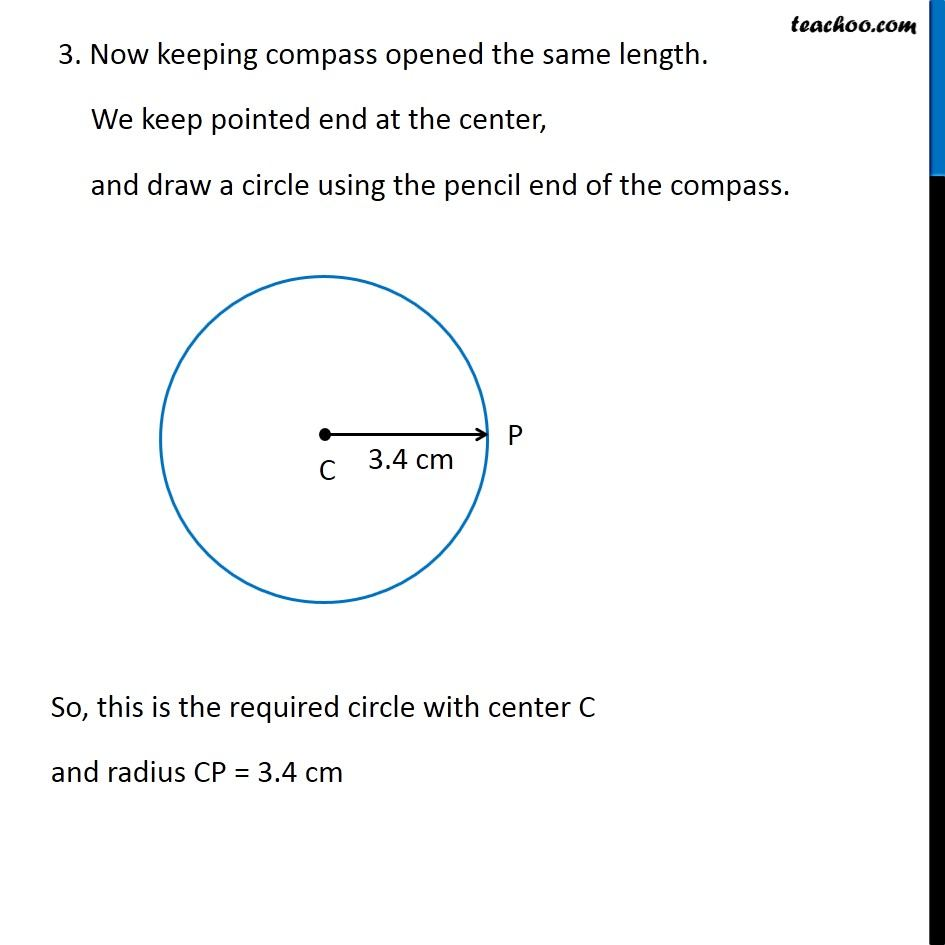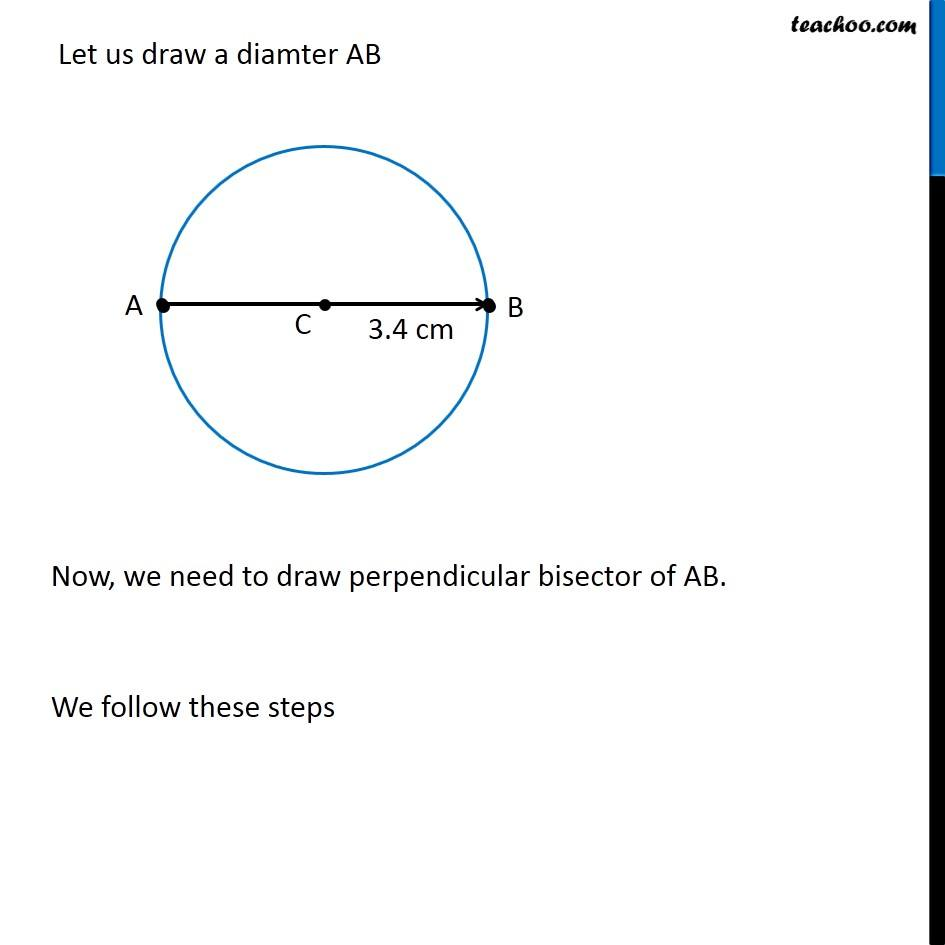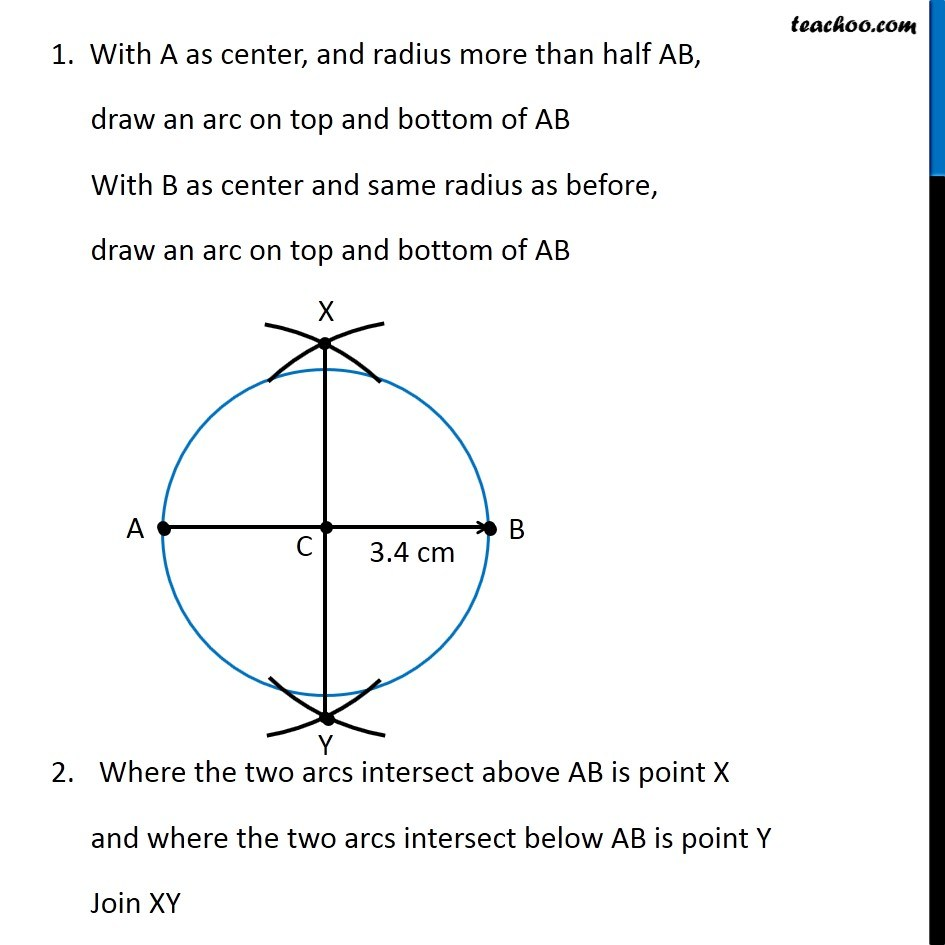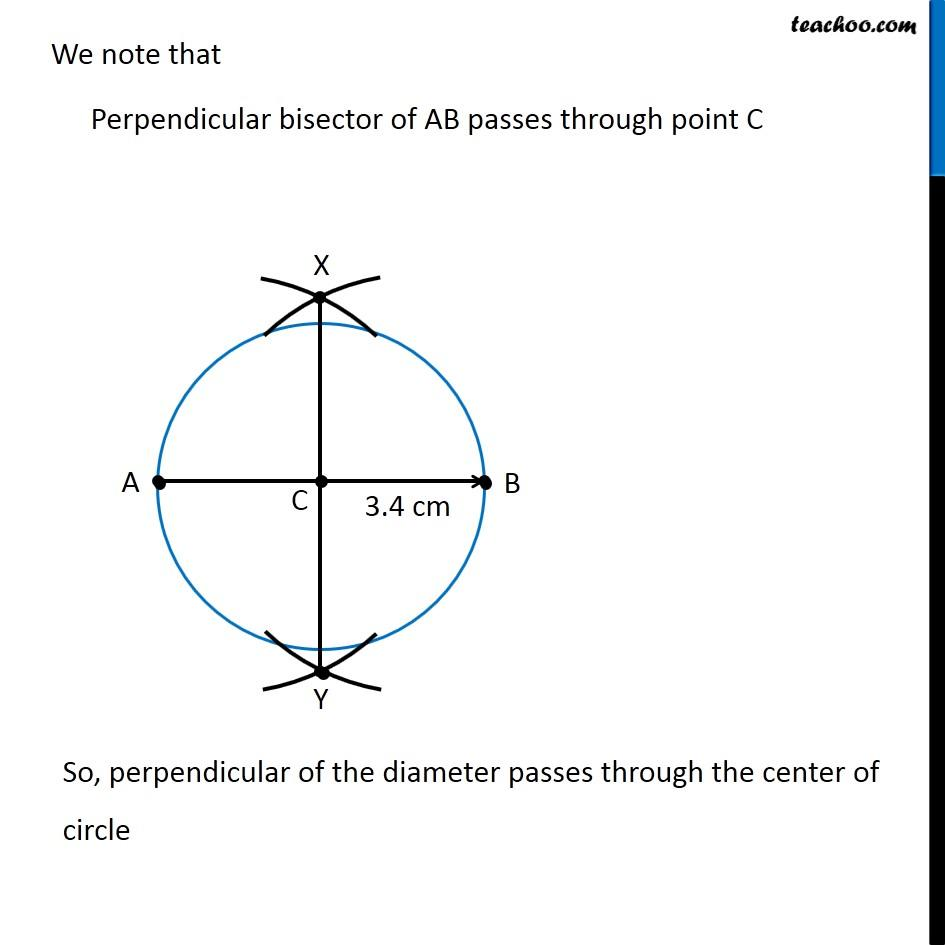Learn in your speed, with individual attention - Teachoo Maths 1-on-1 Class

### Transcript

Question 7 Repeat Question 6, (𝐴𝐵) ̅ happens to be a diameter. Question 6 was Draw a circle with centre C and radius 3.4 cm. Draw any chord (𝐴𝐵) ̅ . Construct the perpendicular bisector of (𝐴𝐵) ̅ and examine if it passes through C. Let’s first draw a circle of radius 3.4 cm We follow these steps 1. Mark point C as center 2. Since radius is 3.4 cm, we measure 3.4 cm using ruler and compass 3. Now keeping compass opened the same length. We keep pointed end at the center, and draw a circle using the pencil end of the compass. So, this is the required circle with center C and radius CP = 3.4 cm Let us draw a diamter AB Now, we need to draw perpendicular bisector of AB. We follow these steps 1. With A as center, and radius more than half AB, draw an arc on top and bottom of AB With B as center and same radius as before, draw an arc on top and bottom of AB Where the two arcs intersect above AB is point X and where the two arcs intersect below AB is point Y Join XY We note that Perpendicular bisector of AB passes through point C So, perpendicular of the diameter passes through the center of circle